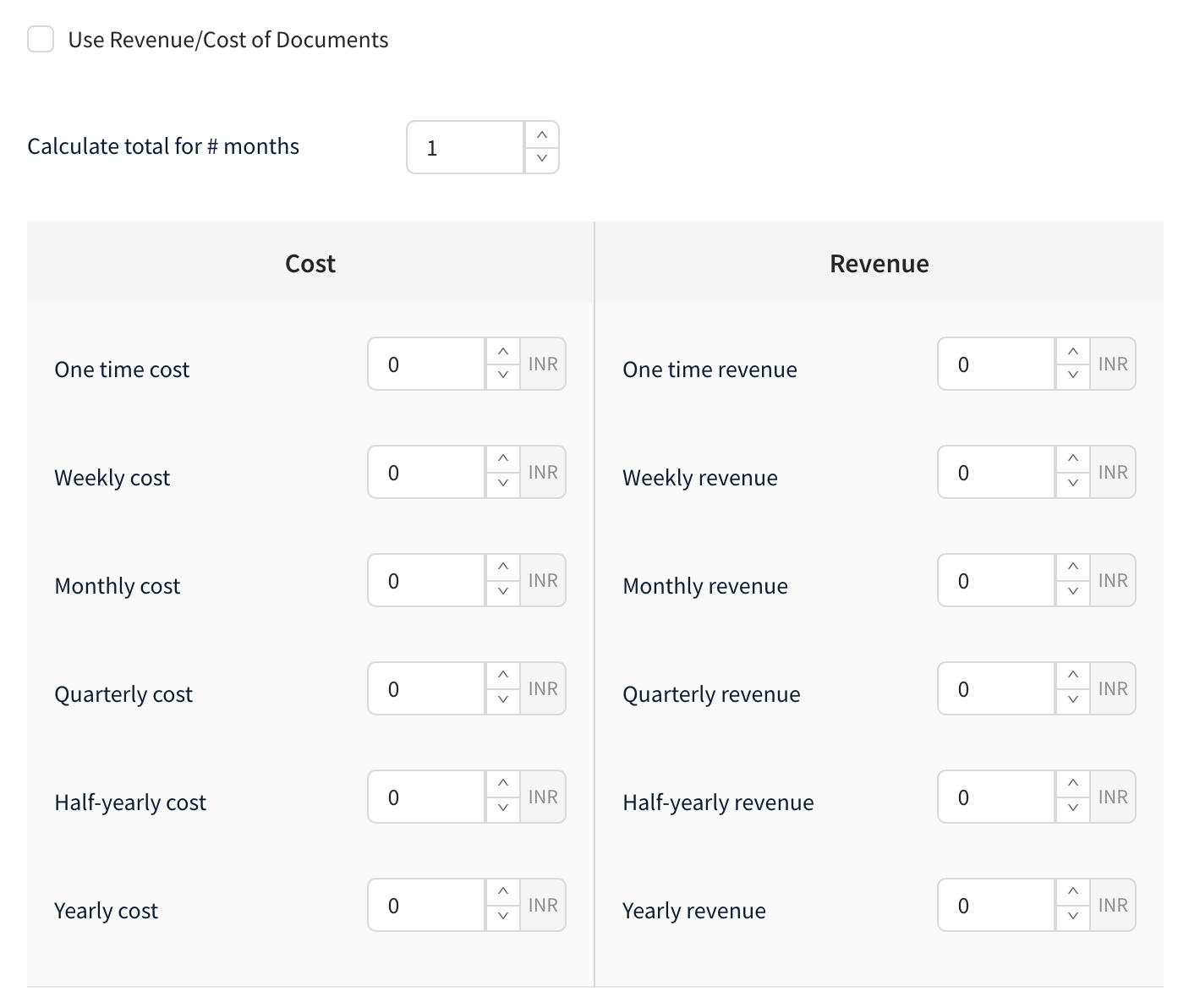If this box is checked, the cost of the opportunity will be automatically taken from the document. If it is unchecked, the cost and revenue can be manually entered into the box given.Note:

(i) You can enter the number of months to calculate the cost and revenue for the opportunity in “Calculate the total for # months

(ii) Calculation of Totals and Margin:

Total Revenue = One-time revenue

+ (Weekly revenue * 4 * Number months to calculate totals)

+ (Monthly revenue * Number months to calculate totals)

+ (Quarterly revenue/3 * Number months to calculate totals)

+ (Half-yearly revenue/6 * Number months to calculate totals)

+ (Yearly revenue/12 * Number months to calculate totals)

Total Cost = One-time cost

+ (Weekly cost * 4 * Number months to calculate totals)

+ (Monthly cost * Number months to calculate totals)

+ (Quarterly cost/3 * Number months to calculate totals)

+ (Half-yearly cost/6 * Number months to calculate totals)

+ (Yearly cost/12 * Number months to calculate totals)

Margin  Value = Total revenue - Total Cost

Margin  Percentage = (Total revenue - Total Cost) / Total revenue

Total Revenue: 1000 + (100 * 4 * 6) + (500 * 6) + (1000/3 *  6) + (5000/6 * 6) + (10000/12 * 6) = 18400

Total Cost: 800 + (80 * 4 * 6) + (400 * 6) + (800/3 *  6) + (4000/6 * 6) + (8000/12 * 6) = 14720

Margin Value: 18400 - 14720 = 3680

Margin percentage: 3680 / 18400 = 20%